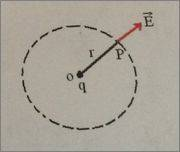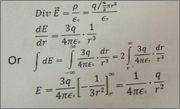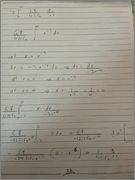# Electric field at point Gauss law

## Homework Statement

We wont to find out the electric field at a point p due to a point charge q placed at o as shown in the figure
[/B]Consider a sphere of radius r passing through the point p. Let the electric field at p be E then by Gauss lawmy problem is how we get the last step? I dont understand how he integrate it.

## The Attempt at a Solution

i tried it and found a solution but not sure if its correct although i got the same result[/B]

sorry that i don't used LATEX but I'm not used with it and i cant write the whole question with LATEX

Doc Al
Mentor
You need to learn the rules for integration (and differentiation) of ##x^n##. Try this: Power Rule

BvU
Homework Helper
Looks as if the power rule is known, but the concept of integration causes difficulties: substitution ##x=r^{-3}## is carried out ok initially (*), but the much better (or rather, the 'right') substitution ##x = r^{-2}##, leading to ##dx =-2\; r^{-3}dr## and hence ##\int r^{-3} dr = -{1\over2}\int dx## is overseen.

(*) Later on, the swap ##\int {x\over r^{-4}}\; dx = {1\over r^{-4}} \int x\; dx## is unforgivable: r is not a constant but depends on x !!

If r is not constant and depends on x. Therefore i cant put the ##r^4## outside the integration. Right??

BvU
Homework Helper
Indeed you can not !

Ok so how to get this solution?
Any idea??
I want the the same result ##E=\frac{q}{4 \pi \epsilon r^2}##

BvU
Homework Helper
OK, we have to make a step back. Your "Consider a sphere of radius r passing through the point p. Let the electric field at p be E then by Gauss law " continues with a picture with formulas that seem to be out of context: at P there is no charge, so div E is zero there !

Where does this snippet come from? It also integrates from ## r=-\infty## to ## r=\infty## which is very weird, since r can't be negative. Then it equates that to ##2\int_0^\infty\ ##, then inserts a ##- {1\over 3 }## from nowhere (re-check the power rule)!

So, stepping back and trying to find the correct path:

Gauss law replaces a surface integral of E by a volume integral of rho. Indeed ##\nabla \vec E = {\rho\over \epsilon_0} ##, but ## \rho \ne q\big /{4\over 3}\pi r^3\ ## ! That is ##\rho## for a uniformly charged sphere with radius r !

In this simple case the volume integral of rho simply yields q. Frome there use rotation symmetry and gauss law to get to E.

Another thing: note that div E is not ## dE\over dr## !

Divergence in spherical coordinates tells us that

for rotational symmetry ##\nabla\cdot\vec E = {1\over r^2}\; {\partial (r^2E_r)\over \partial r}##

(
as you can check: div E = 0 for the desired result for E

and conversely: with rotational symmetry, Er2 is constant outside the area where charge is present.​
).

 forgot the divergence link, inserted it.

Doc Al
Mentor
Ok so how to get this solution?
Any idea??
Sure. Learn how to integrate ##x^n##; in particular, ##\frac{1}{x^3} = x^{-3}##. (No fancy substitutions needed.)

Sure. Learn how to integrate ##x^n##; in particular, ##\frac{1}{x^3} = x^{-3}##. (No fancy substitutions needed.)

I know how to use the power rule.
If you see my solution ;)

@BvU
Thank you for your help. Looks like this question is a mess. The whole question is wrong
I will now use your information and maybe i will get a right solution.

Doc Al
Mentor
I know how to use the power rule.
If you see my solution
Then why did you say the following?
my problem is how we get the last step? I dont understand how he integrate it.
The last step was integrating 1/x3.

I guess I missed something. (Sorry about that!)

Yes thats right but if you try to integrate the last step you will not get the same result. Therefore i said that i dont understand it. But as BvU told me the whole question has mistakes.

BvU
Homework Helper
So is the picture with the formulas from a different exercise ? Or did you do a part of the solution in TeX and then switch to handwritten ?

No the two pictures ( figure and Tex ) are from exercise. Just the handwriting is mine. But the exercise it is not from one source cause the exercise has the soltuion from a screenshot. It has text and image ( the soultion and the figure ) are screenshots.

BvU